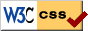# Hermitian Forms Meet Several Complex Variables: Changes

July 23rd 2020 edition:

1. On page 57, $\frac{m-n}{2}$ should be $\max \{ \frac{m-n}{2} , 0 \}$
2. Theorem 1.2.7 requires the hypothesis that $f^{-1}(0)$ has no limit points on $U' \times \partial D$.
3. Mention that $\beta$ are the rows and $\alpha$ columns in section 2.3
4. More explicitly give the computation in Example 2.3.1.
5. Fix some typos in equation 2.20, the bars in second line should go all the way across and $\varphi(0) = p$ just above.
6. Minor spelling, style, typo fixes.

April 8th 2014 edition:

1. Update references, fix reference labels.
2. Fix the Cauchy estimate inequality, it is only the point, not the norm on the left unless a smaller set would be taken.
3. Slightly update the note in the beginning.

February 28th 2011 edition:

1. Include local parametrization theorem for varieties without proof, and add exercise related to it.
2. Fix statement of Theorem 1.2.7.
3. Fix a number of typos found by Montgomery Taylor, thanks!
4. Many minor grammar and typo fixes.

December 2nd 2010 edition:

1. Include more detail in the proofs and improve exposition of the theorems in 2.4 and 2.6
2. Add exercise in 1.4 about parametrization by discs which we actually use later.
3. Minor clarifications.
4. Many minor grammar and typo fixes.

July 15th 2010 edition:

1. Fixed up hyperlinks, which are now underlined as well and have larger targets to click on.
2. Added a bunch of references, more will come in the future sometime.
3. Added some clarifications in places.
4. Lots of spelling, grammar, and stylistic fixes.
5. Using newest texlive so producing PDF 1.5.
6. Using the microtype package with pdflatex to use microtypography. It really looks wonderful and pdflatex does all the work for you.

May 11th 2010 edition:

First version